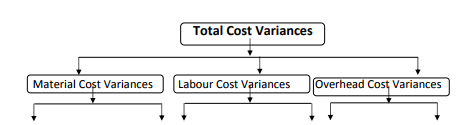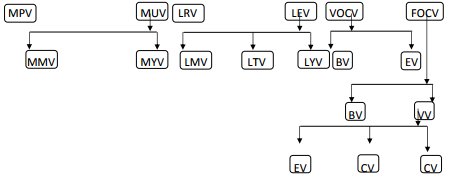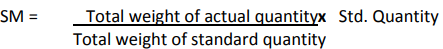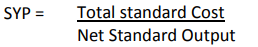Courses

# Types of Variances - Standard Costing, Cost Management B Com Notes | EduRev

## B Com : Types of Variances - Standard Costing, Cost Management B Com Notes | EduRev

The document Types of Variances - Standard Costing, Cost Management B Com Notes | EduRev is a part of the B Com Course Cost Management.
All you need of B Com at this link: B Com

Types of Variances:

Initially, standards for all elements of costs should be set and then the actual cost should be compared with the standard costs to obtain the variances. Some deviations are found when actual performances are recorded and compared with the standard set. These deviations are known as variances.

"A variance is the difference between a standard cost and the comparable actual cost incurred during a period"  ‐ C.I.M.A. London

Variances are classified on the basis of:

1) On the basis of control

2) On the basis of profitability

3) On the basis of elements of cost

(1) On the basis of control: On the basis of control, variance may be classified as controllable variance and uncontrollable variance.

(2) On the basis of profitability: With regard to the profitability or effect, variance may be classified into two:
(i) favourable variance and
(ii) unfavourable variance.

These are also known as credit and debit variance or negative and positive variances.

(3) On the basis of elements of cost: Though different types of  variances can be calculated, their use may not be much useful. Variance calculated on the basis of different elements of cost. They are as follows:

Total Cost Variance is a difference between the standard cost value of the output achieved in a period and the total cost incurred.Material Variances (MV): These variances include Material Cost Variances, Material Price Variances, Material Usage Variances, Material Mix Variances and Material Yield Variances.

(1) Material Cost Variances (MCV): It is the difference between the standard cost of material specified for the output achieved and the actual cost of direct materials used.

MCV =   (Std. Quantity x Std. Price) ‐ (Actual Quantity x Actual Price)

(SQ x SP) ‐ (AQ x AP)

(2) Material Price Variances (MPV): It is that portion of the material cost variance which is due to the difference between the standard price specified and the actual price paid.

MPV =   Actual Quantity (Std. Price ‐ Actual Price)

AQ (SP x AP)

Where, Price = Rate

(3) Material Usage Variances (MUV): Material usage variance is a part of Direct Material Cost Variance. MUV is determined by difference found between the standard quantity and the use of actual quantity. Later, the difference found is multiplied by the standard price.

MUV =   Standard Price (Std. Quantity ‐ Actual Quantity)

SP (SQ ‐ AQ)

(4) Material Mix Variances (MMV): It is that portion of direct material usage variance which is the difference between the actual quantities of elements used in a mixture at a standard price and the total quantity of elements used at the weighted average price per unit of element as shown by the standard cost sheet.

MMV =   Standard Price (Std. Mix ‐ Actual Mix)

SP (SM ‐ AM)Note: When the actual weight of quantity and the standard weight of quantity differ from each other, this formula is used to find new quantity.

(5) Material Yield Variances (MYV): This is "that portion of the direct materials usage variances which is due to the difference between standard yield specified and the actual yield obtained.

MYV =   Standard Yield Price (Std. Yield ‐ Actual Yield)

SYP (SY ‐ AY)Note: When the actual weight of quantity and the standard weight of quantity differ from each other, this formula is used to find new quantity.

Labour Variances (LV): Labour variances occur because of the difference in actual rates and standard rates of labour and the variation in actual time taken by labours and the standard time allotted to them for doing a job. These variances include Labour Cost Variances, Labour Rate Variances, Labour Time or Efficiency Variances, Labour Idle Time Variances, Labour Mix Variances.

(1) Labour Cost Variances (LCV): This is the difference between the standard direct labour cost and the actual direct labour cost incurred for the production achieved.

LCV =   (Std. Time x Std. Rate) ‐ (Actual Time x Actual Rate)

(ST X SR) ‐ (AT x AR)

(2) Labour Rate Variances (LRV): This is that portion of the labour cost variance which is due to the difference between the standard rate specified and the actual rate paid.

LRV =   Actual Time (Std. Rate ‐ Actual Rate)

AT (SR ‐ AR)

Note: Actual Time = Actual Hours, Std. Rate = Std. Wage Rate

(3) Labour Time (Efficiency) Variances: (LTV/LEV): It is defined as the difference between the standard hours (Time) for the actual production achieved and the hours actually worked, valued at the standard labour rate.

LTV =   Standard Rate (Std. Time ‐ Actual Time)

SR (ST ‐ AT)

(4) Idle Time Variance (ITV): ITV comes up because of idle time of workers on account of abnormal causes. The wages paid for the time during which the workers remained idle due to causes like strikes, breakdown on plant, etc. are treated as idle time variances.

ITV =   Idle Time x Standard Rate

IT x SR

(5) Labour Mix Variance / Gang Composition Variance (LMV): It occurs only when more than one grade of workers is employed and the composition of actual grade of workers differs from those specified.

Std. Time x (Revised Std. Time ‐ Actual Time)

ST x (RST ‐ AT)

Overhead Variances (OV): Overhead is the aggregate of indirect materials, indirect labour and indirect expenses. Analysis of overhead variances is different from that of direct material and direct labour variances by two reasons.

(1) It is difficult to establish Standard overhead rate for fixed overhead because changes in the volume of output will affect the standard overhead rate even if there is no change in the amount of fixed overhead cost.

(2) For computing overhead variances, there are quite a few terminological options and methods.

The overhead variances include fixed overhead variances and variable overhead variances. Moreover, further analysis of overhead variances is also possible according as the available source information. It is significant to know at the beginning that the overhead variance is not anything but under or over‐ absorption of the overhead.

(a) Variable Overhead Cost Variance (VCOV): VCOV is the difference between the standard variable overhead cost for production and the actual variable cost incurred during the period.

VCOV =   (Std. hours for actual Output x Std. variable overhead rate) ‐  Actual overhead cost

Absorbed V. O. ‐ Actual V. O.

(1) Variable Overhead Expenditure Variance (VOEV): VOEV is known as spending variance or 'Budget Variance'. This variance arises due to the difference between standard variable overhead allowed and actual variable overhead incurred.

VCOV =   (Std. Variable Overhead Rate x Actual Hours)  ‐  Actual overhead cost

Standard V. O. ‐ Actual V. O.

(2) Variable Overhead Efficiency Variance (VOEV): VOEV can occur due to the difference between standard hours allowed for actual output and actual hours

VOEV =   (Std. Variable for actual output  ‐  Actual hours) x Std. Variable overhead rate

Absorbed V. O. ‐ Standard V. O

Check = V. O. Expenditure Variance + V. O. Efficiency Variance

(b) Fixed Overhead Cost Variances (FOCV): FOCV is the difference between standard fixed overhead cost for actual output and actual fixed overhead.

FOCV =   (Std. hours for actual output x  Std. F. O. Rate) ‐ Actual F. O.

(1) Fixed Overhead Expenditure Variances (FOEV): This is known as spending variance or Budget Variance. It arises due to the difference between budgeted fixed overhead and actual fixed overhead.

(2) Fixed Overhead Volume Variances (FOVV): It is known as that portion of overhead variance which arises due to the difference between standard cost of overhead absorbed by actual production and the standard allowance for that output.

FOVV =   (Std. Time for Actual Output ‐ Budgeted Time) x Std. Rate

(i) Efficiency Variances (EV): It classifies that portion of volume variance which reflects the increased or reduced output arising from efficiency above or below the standard which is expected.

EV =   (Std. Time for Actual Output ‐ Actual Time) x Std. Rate

(ii) Capacity Variances (CV): It classifies that portion of the volume variance which is caused by functioning at higher or lower capacity usage than the standard. It is affected by the factors like strikes, power failure, over demand etc.

CV = (Actual Time Worked ‐ Budgeted Time) x Std. Rate Std.

Note: Actual Time = Actual Hours

(iii) Calendar Variances (CV): It classifies that portion of the volume variance which is caused by the difference between the number of working days in the budget period and the number of actual working days in the period to which the budget is applied.
This variance arises only in exceptional circumstances because normal holidays are taken into account while laying down the standard.

CV = Actual No. of Working Days ‐ Std. No. of Working Days) x Std. Rate per Day

(Revised Budgeted Time ‐ Budgeted Time) x Std. Rate per Time

Offer running on EduRev: Apply code STAYHOME200 to get INR 200 off on our premium plan EduRev Infinity!

## Cost Management

52 videos|51 docs

,

,

,

,

,

,

,

,

,

,

,

,

,

,

,

,

,

,

,

,

,

,

,

,

;